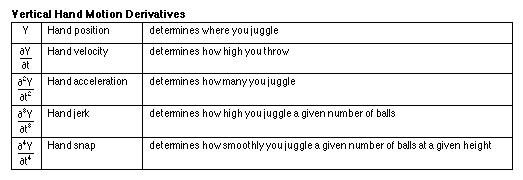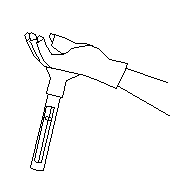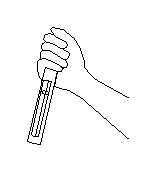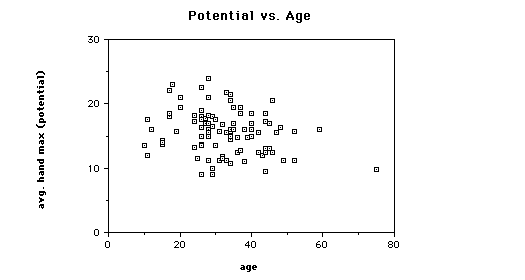## How Many Objects Can Be Juggled

### Jack Kalvan7/24/97

I hate to break it to you aspiring numbers jugglers, but no human will ever juggle 100 balls. Only a handful of people have reached a level to throw eleven or twelve objects into the air, and so far, not for more than a few seconds. No one has even come close to juggling 13 balls. But is this within the realm of human possibility?

Hand speed is one of the main factors that limit the number of objects one can juggle. (The other main factors being accuracy of throws and having long enough arms and enough space in the air for the juggling pattern.) I decided to find out if anyone has the hand speed necessary to juggle 13 or more balls. So I designed an experiment to measure the theoretical human juggling limits - given the acceleration of the hands.

To write the necessary equations, I define the following variables:

b = number of balls
h = number of hands
f = flight time of a ball from throw to catch
tau = time between throws from the same hand
Vv = vertical throw velocity
g = acceleration due to gravity = 9.81 m/s/s
r = "dwell ratio" or fraction of time a hand is holding a ball. My tests show r is usually about 2/3.
omega = average number of balls in flight per arc

One can also think of r as the average number of balls in a hand while juggling. omega can be expressed as the number of balls per hand minus the balls held in the hand: omega = (b / h) - r .

omega is also equal to the time that balls are in flight divided by how often they are thrown: omega = f / tau .

To simplify my analysis, I will assume balls are thrown and caught at the same height. Newtonian physics tells us the flight time of a ball, f = 2 Vv / g . Substituting this equations for f into the second equation for omega gives omega = 2 Vv / g / tau .

Since g is a constant, we see that omega is proportional to the throwing velocity of the hand divided by the time between throws. This means the number of balls in the air while juggling is closely related to the acceleration of the hand. Although a juggler's hands do not necessarily accelerate smoothly, the number of balls one can get into the air is approximately proportional to the maximum acceleration of one's hands .

I figured if I measure the maximum acceleration of a juggler's hands with a simple accelerometer, I could roughly calculate the juggler's maximum value for omega. And substituting this value into the equation, b / h = omega + r, gives an approximation of the maximum number of balls one can theoretically juggle. Remember, this maximum number of balls is calculated only from the speed a juggler can potentially throw balls into the air. It does not take into account accuracy of throws or the possibility of collisions.

Since the number of balls juggled is proportional to hand acceleration, a corollary is that the height of your juggling pattern is not related to your hand acceleration. For example, if you juggle 5 balls high, you have about the same hand acceleration as if you juggle them low. The difference is that to juggle high, you accelerate for a longer time and therefore have longer hand motion and a higher throwing velocity.

I believe the following chart describes how the derivatives of the vertical hand motion relate to juggling:#### The JugglemeterThis simple device measures the hand acceleration. A small mass is connected to a spring inside a tube. When the hand accelerates the device (by shaking it up and down), two opposing forces act on the mass: the acceleration force (force = mass * acceleration) and the spring force (force = spring stiffness * distance stretched). These forces are in equilibrium when the spring is stretched. A marker measures the maximum distance the spring was stretched.

Since the mass and spring stiffness are constant, the maximum acceleration is proportional to this distance. The distance the spring stretches is therefore proportional to the number of balls potentially juggled.

#### Jugglemeter CalibrationTo calibrate the device, I attached it to the back of a glove. This method worked well for low numbers of balls but the glove and the weight of the jugglemeter made juggling more difficult. Also imperfect juggling led to artificially high readings because more acceleration is needed to catch inaccurate throws.To calibrate and test the jugglemeter for higher numbers of balls, I found it was easiest to first juggle a number of balls for long enough to get the feel of it. Then, I'd drop the balls, pick up the jugglemeter, and reproduce the same hand motion while holding it. This process is not extremely accurate since it relies on the ability to reproduce the same hand motion as juggling but with the balls removed. However, after tests with many jugglers and up to 10 balls, and extrapolating the results, I feel confident that my calibration error is less than 10%.

Each juggler I tested repeated the calibration test with an amount of balls that they could juggle comfortably, to convince themselves that the meter calibration was fairly accurate. Some people were unable to simulate the proper hand motion without actually juggling the balls. On the other hand, many were able to reproduce the proper hand motion, and after pretending to juggle 5 balls, were shocked to see the meter reading "5". "How does it know?!!"

To find the maximum potential of each subject, I told them to shake the jugglemeter as hard and fast as they could, for a few seconds, in an up-and-down motion. (This was the most interesting part for spectators.) I measured the maximum with each hand and allowed one retry if they thought they could do better.

#### Summary of Test Results

I tested over 100 people ranging from non-jugglers to some of the best jugglers in the world. Every one of the test subjects had the hand speed to juggle 9 balls. The average was about 16 balls, and the highest recorded was about 24 balls. Hand speed did tend to increase slightly with juggling ability. Those who said they had flashed 9 or more balls averaged about 18.3, while those who had never flashed 5 balls averaged about 14.4.

Comparing subjects with comparable juggling ability: hand speed averaged highest for people around 18 years old; there was a small decrease in hand speed with age; and males tended to get slightly higher readings than females.

Often there was a difference between the two hands and many were surprised to find they did better with their "bad" hand.

I suspect that some of the test subjects were not trying as hard as they could have. Also I suspect some could have done better by relaxing their arms more -- it helped me.#### Conclusions

Every person I tested demonstrated the hand acceleration needed to juggle a smooth 9 ball cascade. Unfortunately, catching wild throws in a messy, inaccurate 9 ball cascade requires much more acceleration than this. And very few of the test subjects have the accuracy to juggle 9 balls smoothly.

By practicing, one's accuracy improves, the demands on one's acceleration are lessened, and the juggling actually requires less and less effort. It requires much less effort to juggle 7 balls well than to juggle 7 balls badly.

Here are some illustrations of how inaccuracy leads to higher acceleration. If one makes a throw too far across and has to move the catching hand twice the usual horizontal distance in the same amount of time, this requires twice as much horizontal acceleration. If one's inaccuracy is in throw height, there is an even more unfortunate relationship. Suppose one hand makes a slightly high throw followed by a slightly low throw, so that the two balls come down at almost the same time. If a throw has to be made in half the normal time, this requires four times the vertical acceleration. If juggling 7 balls, this correction would probably be beyond human capabilities.

I believe that eventually someone will juggle 13 balls, and flashing 15 doesn't seem too unlikely. But how can anyone get to juggling 13 balls smoothly without wasting many years trying to juggle 13 balls badly? It will probably require new training techniques; for example a technique that allows the juggler to practice throwing balls accurately at 15 ball juggling speed, without worrying about catching them and picking them up every few seconds. But that's another paper.

The Human Limits / Juggling Papers / jis@juggling.org